Custom SearchSUMMARY With the completion of this chapter you now have all the building blocks for electrical circuits. The subjects covered from this point on will be based upon the concepts and relationships that you have learned. The following summary is a brief review of the subjects covered in this chapter. INDUCTANCE IN AC CIRCUITS - An inductor in an ac circuit opposes any change in current flow just as it does in a dc circuit. PHASE RELATIONSHIPS OF AN INDUCTOR - The current lags the voltage by 90 in an inductor (ELI). INDUCTIVE REACTANCE - The opposition an inductor offers to ac is called inductive reactance. It will increase if there is an increase in frequency or an increase in inductance. The symbol is XL, and the formula is XL = 2pfL.CAPACITANCE IN AC CIRCUITS - A capacitor in an ac circuit opposes any change in voltage just as it does in a dc circuit. PHASE RELATIONSHIPS OF A CAPACITOR - The current leads the voltage by 90 in a capacitor (ICE). CAPACITIVE REACTANCE - The opposition a capacitor offers to ac is called capacitive reactance. Capacitive reactance will decrease if there is an increase in frequency or an increase in capacitance. The symbol is XC and the formula is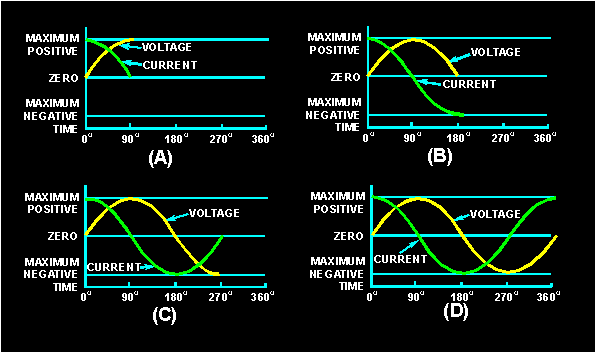TOTAL REACTANCE - The total reactance of a series ac circuit is determined by the formula X = XL - XC or X = XC - X L. The total reactance in a series circuit is either capacitive or inductive depending upon the largest value of XC and XL. In a parallel circuit the reactance is determined by where IX = IC - IL or IX = IL - IC. The reactance in a parallel circuit is either capacitive or inductive depending upon the largest value of IL and IC.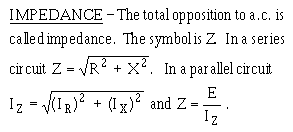PHASE ANGLE - The number of degrees that current leads or lags voltage in an ac circuit is called the phase angle. The symbol is q. OHM'S LAW FORMULAS FOR AC - The formulas derived for Ohm's law used in ac are: E = IZ and I = E/Z. TRUE POWER - The power dissipated across the resistance in an ac circuit is called true power. It is measured in watts and the formula is: True Power = (IR)2R. REACTIVE POWER - The power returned to the source by the reactive elements of the circuit is called reactive power. It is measured in volt-amperes reactive (var). The formula is: Reactive Power = (IX)2X. APPARENT POWER - The power that appears to the source because of circuit impedance is called apparent power. It is the combination of true power and reactive power and is measured in volt-amperes (VA). The formulas are: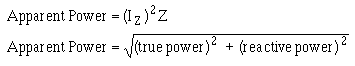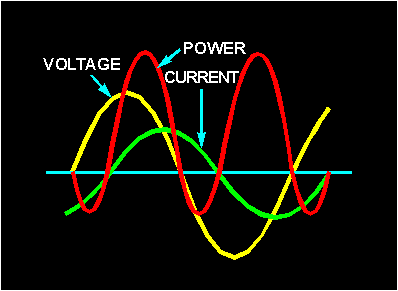POWER FACTOR - The portion of the apparent power dissipated in a circuit is called the power factor of the circuit. It can be expressed as a decimal or a percentage. The formulas for power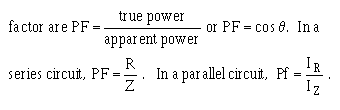POWER FACTOR CORRECTION - To reduce losses in a circuit the power factor should be as close to unity or 100% as possible. This is done by adding capacitive reactance to a circuit when the total reactance is inductive. If the total reactance is capacitive, inductive reactance is added in the circuit.Integrated Publishing, Inc. - A (SDVOSB) Service Disabled Veteran Owned Small Business